# Test: Coordinate Geometry- 4

## 15 Questions MCQ Test General Aptitude for GATE | Test: Coordinate Geometry- 4

Description
This mock test of Test: Coordinate Geometry- 4 for GATE helps you for every GATE entrance exam. This contains 15 Multiple Choice Questions for GATE Test: Coordinate Geometry- 4 (mcq) to study with solutions a complete question bank. The solved questions answers in this Test: Coordinate Geometry- 4 quiz give you a good mix of easy questions and tough questions. GATE students definitely take this Test: Coordinate Geometry- 4 exercise for a better result in the exam. You can find other Test: Coordinate Geometry- 4 extra questions, long questions & short questions for GATE on EduRev as well by searching above.
QUESTION: 1

### The point on the x – axis which is equidistant from the points (2, – 5) and ( – 2, 9) is

Solution: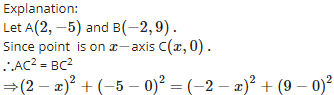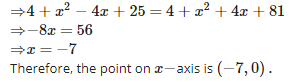QUESTION: 2

### The distance of the point ( – 5, 12) from the y – axis is

Solution:

Explanation:The distance of any point from y−axis is its abscissa. Therefore, the required distance is 5 units.

QUESTION: 3

### The point where the medians of a triangle meet is called the ________ of the triangle

Solution:

Explanation:

The point where three medians of a triangle meet is called the centroid of the triangle.it is the centre of gravity of the triangle. it divides the median in the ratio 2 :1

QUESTION: 4

If the co – ordinates of a point are ( – 5, 11), then its abscissa is

Solution:

Explanation:

Since x−coordinate of a point is called abscissa.Therefore, abscissa is −5.

QUESTION: 5

If the end points of a diameter of a circle are ( – 4, – 3) and (2, 7), then the co – ordinates of the centre are

Solution:

Explanation:

Let the coordinates of centre O be (x,y). The end points of diameter of the circle are A (- 4, - 3) and B(2, 7).

Since centre is the midpoint of diameter.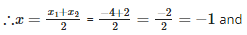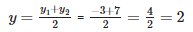Therefore, the coordinates of the centre O is (−1,2).

QUESTION: 6

The centroid of a triangle divides the median in the ratio

Solution:

Explanation:

The centroid of a triangle is the centre of the triangle which is the point of intersection of all the three medians of the triangle and divides the median in the ratio 2 : 1The median is a line drawn from the mid-point of a side to the opposite vertix

QUESTION: 7

The abscissa of any point on the y – axis is

Solution:

Explanation:Since coordinates of any point on y−axis is (0,y).Therefore, abscissa is 0.

QUESTION: 8

The point on the y – axis which is equidistant from the points (6, 5) and ( – 4, 3) is

Solution: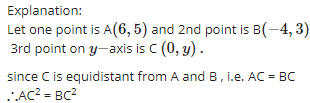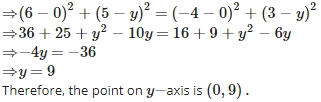QUESTION: 9

If one end of a diameter of a circle is (4, 6) and the centre is ( – 4, 7), then the other end is

Solution:

Explanation:

one end of a diameter is A(4 , 6) and the centre is O ( - 4 , 7) .... ( Given)

Let the other end be B (x,y)
Since centre is the mid-point of diameter of the circle.

therefore coordinates of centre O are  x = (4 + x) / 2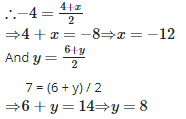Therefore, the required coordinates of other end of the diameter are (−12,8).

QUESTION: 10

The co – ordinates of the mid – point of the line segment joining the points ( – 2, 3) and (4, – 5) are

Solution:

Explanation:

Let the coordinates of midpoint C(x,y)(x,y) of the line segment joining the points A(−2,3) and B(4,−5).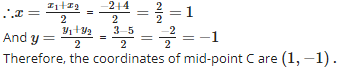QUESTION: 11

If the mid – point of the line segment joining the points (a, b – 2) and ( – 2, 4) is (2, – 3), then the values of ‘a’ and ‘b’ are

Solution:

Explanation:

Let the coordinates of midpoint O(2,−3) is equidistance from the points A(a,b−2) and B(−2,4).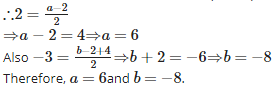QUESTION: 12

The abscissa of any point on the x – axis is

Solution:

Explanation:Since coordinates of any point on x−axis is (x,0).Therefore, abscissa is x.

QUESTION: 13

Find the value of ‘k’, if the point (0, 2) is equidistant from the points (3, k) and (k, 5)

Solution:

Explanation:

Let point C (0, 2) is equidistant fromthe points A(3,k) and B(k,5).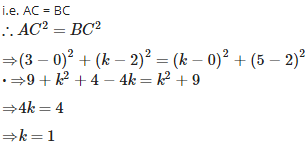QUESTION: 14

The points A( – 1, 0), B(3, 1), C(2, 2) and D( – 2, 1) are the vertices of a

Solution:

Explanation:

Given: The points A( – 1, 0), B(3, 1), C(2, 2) and D( – 2, 1)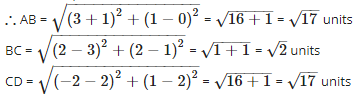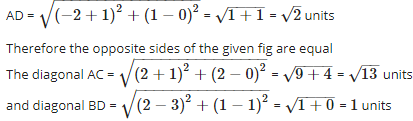Therefore diagonals AC and BD are not equal
Since, opposite sides of the given fig are equal and both diagonals are not equal.
Therefore, the given figure (Quadrilateral) is a parallelogram.

QUESTION: 15

If one end of a diameter of a circle is (2, 3) and the centre is ( – 2, 5), then the other end is

Solution:

Explanation:

Let the coordinates of the other end be B(x2,y2).

One end of the diameter is A (2, 3) and the centre is O(−2,5).
Since the centre is midpoint of the diameter of the circle.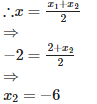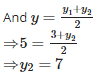Therefore, the coordinates of other end of the diameter are (−6,7).